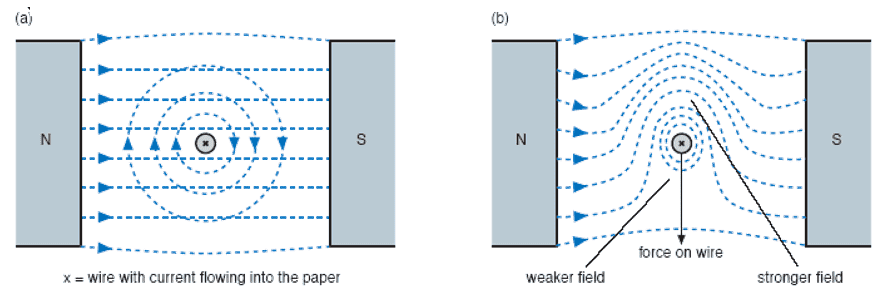# Questions about a Magnetic forces due to an unbalanced field, and about Newton's 3rd law

songokuI understand how the weaker and stronger field occurs but I don't understand why the force on the wire is directed from stronger to weaker field. I mean why not from weaker to stronger?

I also want to ask about Newton's 3rd law in this case. Newton's 3rd law states that for every action there will be equal and opposite reaction. So let say the force on wire in the picture as "action", what will be the "reaction"? The force on the wire is due to the (unbalanced) field so the "reaction" will be the force on (unbalanced) field by the wire?

Thanks

Gold Member
2022 Award
The force on the wire is due to the current running through it. If you make the field of the magnet ##\vec{B}=B \vec{e}_x## then ##\vec{j}=-j \vec{e}_z## and the force per unit volume of the wire is ##\vec{f}=\vec{j} \times \vec{B}=-j B \vec{e}_y##. I don't know, what this has to do with an "unbalanced field" (whatever this might be).

Last edited:
•songoku and etotheipi
songoku
I am really sorry, I don't understand the notation used.

The force on the wire is due to the current running through it. If you make the field of the magnet ##\vec{B}=B \vec{e}_x## then ##\vec{j}=-j \vec{e}_z##

Do you mean ##\vec{B}## is the magnetic field of the magnet (which is to the right based on the picture)?

And what is ##\vec{j}## ?

I don't know, what this has to do with an "unbalanced field" (whatever this might be).
By unbalanced field, I mean there is stronger and weaker region of magnetic field (in picture (b)).

I was taught that the magnetic force occurs due to interaction between 2 magnetic fields, one is from the magnet and one is produced by the current flowing through the wire. This interaction will create unbalanced field, one region is stronger (upper part of wire in picture (b) ) and one region is weaker (lower part of wire in picture (b) ). This stronger and weaker field will give rise to magnetic force acting on the wire, which is directed from stronger field to weaker field (downwards based on picture (b) )

Thanks

Gold Member
2022 Award
Two magnetic fields do not interact (in classical electrodynamics). The total field is just the sum of the fields of the permanent magnet (which I assumed to be approximately constant as given in the picture) and the field due to the current through the wire, and ##\vec{j}## is the current density in the wire. The total force is
$$\vec{F}=V \vec{f}=-A l j B \vec{e}_y=-l I B \vec{e}_y,$$
where ##l## is the length of the wire and ##A## its cross-sectional area.

•songoku and etotheipi
I also want to ask about Newton's 3rd law in this case. Newton's 3rd law states that for every action there will be equal and opposite reaction. So let say the force on wire in the picture as "action", what will be the "reaction"?

The wire also exerts a force on the magnet!

•songoku, berkeman and vanhees71
Mentor
I understand how the weaker and stronger field occurs but I don't understand why the force on the wire is directed from stronger to weaker field. I mean why not from weaker to stronger?
You can think of this in terms of energy. The energy in the field is proportional to the square of the B field. If the wire moves in the direction of the weaker field then that will increase the weak field and decrease the strong field. Because the energy is proportional to the square of the field the energy reduction of the strong field will be larger than the energy increase of the weak field. This implies a force in that direction which can be used to do work equal to the net reduction in energy.

•songoku and vanhees71
songoku
The wire also exerts a force on the magnet!
Let say the force on the wire is F, so there will be upwards force of magnitude F on right magnet and there will also be upwards force of magnitude F on left magnet?

Thanks

Mentor
Let say the force on the wire is F, so there will be upwards force of magnitude F on right magnet and there will also be upwards force of magnitude F on left magnet?
If there were then you would have a reactionless drive. So no.

•songoku
songoku
If there were then you would have a reactionless drive. So no.
Or maybe the force on left and right magnet should be F/2 upwards for each?

Thanks

$$\vec{F}_{\text{mag}}=\int_{V} \mathrm{d}^3 x \vec{\nabla} (\vec{M} \cdot \vec{B}_{\text{wire}}),$$
•